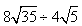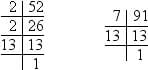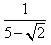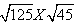Test: Real Numbers

# Test: Real Numbers

Test Description

## 20 Questions MCQ Test Advance Learner Course: Mathematics (Maths) Class 8 | Test: Real Numbers

Test: Real Numbers for Class 9 2022 is part of Advance Learner Course: Mathematics (Maths) Class 8 preparation. The Test: Real Numbers questions and answers have been prepared according to the Class 9 exam syllabus.The Test: Real Numbers MCQs are made for Class 9 2022 Exam. Find important definitions, questions, notes, meanings, examples, exercises, MCQs and online tests for Test: Real Numbers below.
Solutions of Test: Real Numbers questions in English are available as part of our Advance Learner Course: Mathematics (Maths) Class 8 for Class 9 & Test: Real Numbers solutions in Hindi for Advance Learner Course: Mathematics (Maths) Class 8 course. Download more important topics, notes, lectures and mock test series for Class 9 Exam by signing up for free. Attempt Test: Real Numbers | 20 questions in 20 minutes | Mock test for Class 9 preparation | Free important questions MCQ to study Advance Learner Course: Mathematics (Maths) Class 8 for Class 9 Exam | Download free PDF with solutions
 1 Crore+ students have signed up on EduRev. Have you?
Test: Real Numbers - Question 1

### Which of the following statement about real numbers is false?

Test: Real Numbers - Question 2

### The decimal fraction in which there are finite number of digits in its decimal part is called

Detailed Solution for Test: Real Numbers - Question 2

A decimal is a number with a decimal point. There are usually numbers to the left and numbers to the right of that decimal point. For example,​ 0.5 and 12.56 are both decimals. Decimals allow us to represent partial amounts of a whole, just like fractions. As a matter of fact, many decimals and fractions are interchangeable so either form can be used.

Test: Real Numbers - Question 3

### If two positive integers p and q can be expressed as p = ab2 and q = a3b; a, b being prime numbers, then LCM (p, q) is

Detailed Solution for Test: Real Numbers - Question 3

Given that, p = ab2 = a × b × b
and q = a3b = a × a × a × b
∴ LCM of p and q = LCM (ab2,a3b) = a × b × b × a × a = a3b2
[Since, LCM is the product of the greatest power of each prime factor involved in the numbers].

Test: Real Numbers - Question 4

If two positive integers a and b are written as a = x3y2 and b = xy3; x, y are prime numbers, then HCF (a, b) is

Detailed Solution for Test: Real Numbers - Question 4

Given that, a =x3y2 = x × x × x × y × y
and b = xy3 = x × y × y × y
∴ HCF of a and b = HCF (x3y2,xy3) = x × y × y = xy
[Since, HCF is the product of the smallest power of each common prime factor involved in the numbers]

Test: Real Numbers - Question 5

SimplifyTest: Real Numbers - Question 6

A forester wants to plant 44 apples tree, 66 banana trees and 110 mango trees in equal rows (in terms of number of trees). Also, he wants to make distinct rows of tree (i.e. only one type of tree in one row). The number of rows (minimum) that required is:

Detailed Solution for Test: Real Numbers - Question 6

In such case, we first need to find the HCF of 44, 66, 110.
44 = 2 x 2 x 11;
66 = 2 x 3 x 11;
110 = 2 x 5 x 11;
HCF = 2*11 = 22.
Then, the required numbers of rows,
= {(44/22)+(66/22)+(110/22)} = 10.

Test: Real Numbers - Question 7

The HCF and LCM of two numbers are 33 and 264 respectively. When the first number is completely divided by 2 the quotient is 33. The other number is:

Detailed Solution for Test: Real Numbers - Question 7

Given HCF = 33 and LCM = 264
First number a = 2(33) = 66
Let the second number be b
Recall the formula, LCM × HCF = a × b
264 × 33 = 66 × b
b =  264 × 33 / 66 = 132
The second number is 132.

Test: Real Numbers - Question 8

What will be the greatest possible length of the planks, if three pieces of timber 42 m, 49 m and 63 m long have to be divided into planks of the same length?

Detailed Solution for Test: Real Numbers - Question 8

The lengths of three pieces of timber are 42m, 49m and 63m respectively.

We have to divide the timber into equal length of planks.

∴ Greatest possible length of each plank = HCF (42, 49, 63)

Prime factorization:

42 = 2 × 3 × 7

49 = 7 × 7

63 = 3 × 3 × 7

∴HCF = Product of the smallest power of each common prime factor involved in the numbers = 7

Hence, the greatest possible length of each plank is 7m.

Test: Real Numbers - Question 9

If the HCF of 65 and 117 is expressible in the form 65m – 117, then the value of m is

Detailed Solution for Test: Real Numbers - Question 9

Find the HCF of 65 and 117,

117 = 1×65 + 52

65 = 1× 52 + 13

52 = 4 ×13 + 0

∴ HCF of 65 and 117 is 13.

65m - 117 = 13

65m = 117+13 = 130

∴m =130/65 = 2

Test: Real Numbers - Question 10

The least number that is divisible by all the numbers from 1 to 10 (both inclusive) is

Detailed Solution for Test: Real Numbers - Question 10

Factors of 1 to 10 numbers
1=1
2=1×2
3=1×3
4=1×2×2
5=1×5
6=1×2×3
7=1×7
8=1×2×2×2
9=1×3×3
10=1×2×5
∴ LCM of number 1 to 10 = LCM (1,2,3,4,5,6,7,8,9,10)
= 1×2×2×2×3×3×5×7 = 2520

Test: Real Numbers - Question 11

What is the greatest possible speed at which a man can walk 52 km and 91 km in an exact number of minutes?

Detailed Solution for Test: Real Numbers - Question 11

Factors of 52 and 91 areso 52 = 2 × 2 × 13

and 91 = 7 × 13

∴ H.C.F. of 52 and 91 = 13

Hence required speed in minutes = 13 km / min.

Test: Real Numbers - Question 12

Pairs of natural numbers whose least common multiple is 78 and the greatest common divisor is 13 are:

Detailed Solution for Test: Real Numbers - Question 12

Let the no.s be a & b,

GCD [ a , b ] = 13;
=> Let a = 13m and b = 13n

Now, LCM [ a , b ] = 78
=> 13m | 78
=> m | 6 and similarly, n | 6; --> Also, m does not divide n

.'. Possible values of (m,n) are :->
--> [ 1 , 6 ] , [ 2 , 3 ]
.'. Possible values for ( a , b ) are :-> [ 13 , 78 ] , [ 26 , 39 ]

Test: Real Numbers - Question 13

Which of the following is a rational number?

Detailed Solution for Test: Real Numbers - Question 13

0 is an integer and all integers are rational numbers.

Test: Real Numbers - Question 14

If two positive integers p and q can be expressed as p = ab2 and q = a3b; a, b being prime numbers, then LCM (p, q) is

Detailed Solution for Test: Real Numbers - Question 14

As per question, we have,
p = ab2 = a × b × b
q = a3b = a × a × a × b
So, their Least Common Multiple (LCM) = a3 × b2

Test: Real Numbers - Question 15

Every real number is

Detailed Solution for Test: Real Numbers - Question 15

Either you can make a fraction from two whole numbers (denominator ≠ 0), thus a rational number. Or you can't, thus irrational.

Test: Real Numbers - Question 16

The largest number which divides 70 and 125, leaving remainders 5 and 8 respectively, is

Detailed Solution for Test: Real Numbers - Question 16

Since, 5 and 8 are the remainders of 70 and 125, respectively. Thus, after subtracting these remainders from the numbers, we have the numbers 65 = (70-5),
117 = (125 – 8), which is divisible by the required number.
Now, required number = HCF of 65,117                                     [for the largest number]
For this, 117 = 65 × 1 + 52 [∵ dividend = divisior × quotient + remainder]
⇒ 65 = 52 × 1 + 13
⇒ 52 = 13 × 4 + 0
∴ HCF = 13
Hence, 13 is the largest number which divides 70 and 125, leaving remainders 5 amnd 8.

Test: Real Numbers - Question 17

Rationalizing Factor isis

Test: Real Numbers - Question 18

The least number that is divisible by all the numbers from 1 to 10 (both inclusive) is

Detailed Solution for Test: Real Numbers - Question 18

We have to find the least number that is divisible by all numbers from 1 to 10.

∴ LCM (1 to 10) = 23 × 32 × 5 × 7 = 2520

Thus, 2520 is the least number that is divisible by every element and is equal to the least common multiple.

Test: Real Numbers - Question 19

SimplifyDetailed Solution for Test: Real Numbers - Question 19

√5×√5×√5 × √5×√3×√3 5×5×3 =75

Test: Real Numbers - Question 20

The least number that is divisible by all the numbers from 1 to 10 (both inclusive) is

Detailed Solution for Test: Real Numbers - Question 20

Factors of 1 to 10 numbers
1=1
2=1×2
3=1×3
4=1×2×2
5=1×5
6=1×2×3
7=1×7
8=1×2×2×2
9=1×3×3
10=1×2×5
∴ LCM of number 1 to 10 = LCM (1,2,3,4,5,6,7,8,9,10)
= 1×2×2×2×3×3×5×7 = 2520

## Advance Learner Course: Mathematics (Maths) Class 8

13 videos|61 docs|24 tests
 Use Code STAYHOME200 and get INR 200 additional OFF Use Coupon Code
Information about Test: Real Numbers Page
In this test you can find the Exam questions for Test: Real Numbers solved & explained in the simplest way possible. Besides giving Questions and answers for Test: Real Numbers, EduRev gives you an ample number of Online tests for practice

## Advance Learner Course: Mathematics (Maths) Class 8

13 videos|61 docs|24 tests